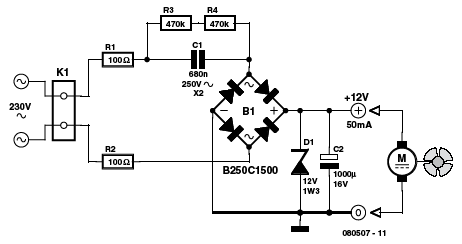Home | Gadget | Fuzzy | Lecturer  | Power  | Transceiver Find more type here... 12V Fan Directly on 230 V Ton Giesberts   This circuit idea is certainly not new, but when it comes to making a trade-off between using a small, short-circuit proof transformer or a capacitive voltage divider (directly from 230 V mains voltage) as the power supply for a fan, it can come in very handy. If forced cooling is an afterthought and the available options are limited then perhaps there is no other choice. At low currents a capacitive divider requires less space than a small, short-circuit proof transformer. R1 and R2 are added to limit the inrush current into power supply capacitor C2 when switching on. Because the maximum rated operating voltage of resistors on hand is often not known, we choose to have two resistors for the current limit. The same is true for the discharge resistors R3 and R4 for C1. If the circuit is connected to a mains plug then it is not allowed that a dangerous voltage remains on the plug, hence  R3 and R4. Capacitor C1 determines the maximum current that can be supplied. Above that maximum the power supply acts as a current source. If the current is less then zener diode D1 limits the maximum voltage and dissipates the remainder of the power. It is best to choose the value of C1 based in the maximum expected current. As a rule of thumb, start with the mains voltage when calculating C1.The 12 V output voltage, the diode forward voltage drops in B1 and the voltage drop across R1 and R2 can be neglected for simplicity. The calculated value is then rounded to the nearest E-12 value. The impedance of the capacitor at 50 Hz is 1 / (2π50C). If, for example, we want to be able to supply 50 mA, then the required impedance is 4600 Ω (230 V/50 mA). The value for the capacitor is then 692 nF. This then becomes 680 nF when rounded. To compensate for mains voltage variationsand the neglected voltage drops you could potentially choose the next higher E-12 value. You could also create the required capacitance with two smaller capacitors. This could also be necessary depending on the shape of the available space. It is best to choose for C1 a type of capacitor that has been designed for mains voltageapplications (an X2 type, for example).